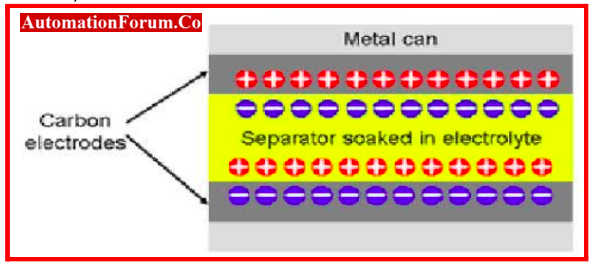# What is a Non-Linear Device? Example & Advantages## Electrical / Electronic device

Electrical or electronic elements are separated into two types based on the characteristics of the current flow when voltage is applied across them:

• Linear Element
• Nonlinear Element

## Linear Element

• When the applied voltage across linear elements varies, the current flow through them also changes linearly.
• Example- Resistor, Inductor & Capacitor

## Characteristics of Linear element

The voltage-current graph of linear element is shown below.

## Non-Linear Element

Non-linear elements are those through which the applied voltage changes without linearly affecting the current flowing through them. The voltage-current graph of the non-linear element is often exponential rather than a straight line like the linear element. This exponential line shows that the relationship between an increase in current and an increase in applied voltage is not linear or homogeneous.

## Non-Linear Devices

Non-linearity can be understood from the response between the input power (or voltage) and the output power. The response shows the output for a given input is non-linear.

### Examples of Non-Linear Devices

The best example of a non-linear device is a diode, PN Junction. All semiconductor devices, including BJT and FET, can be regarded as examples of non-linear devices.

### Properties of Non-Linear Devices

1. Non-linear components typically do not adhere to Ohm’s Law.
2. The voltage current relationship is non-linear.
3. Although the voltage current graph is not always a straight line, it may of exponential form.
4. It’s possible that the current flow through the non-linear parts is not constant.

### Where do the non-linear devices are used?

• Controlling the flow of current through a non-linear device is its primary purpose. Because of this, the majority of the linear components are controlled by current.
• Anyhow, non-linear elements are utilized in applications for frequency control, rectification, current source, and current control.

### Some of the Non-Linear Devices and circuits

#### Surge Protection Circuit

• Surge protectors often combine non-linear components like MOVs and BBD’s (bidirectional break-over diodes) with inductors, capacitors, and other non-linear components. The world of electronic components has seen the emergence of electrical double-layer capacitors, sometimes known as supercapacitors or ultra-capacitors.
• For the same volume as an electrolytic or film capacitor, these typically have a million times higher capacitance. However, their DC voltage ratings, which are in the range of (2-4) V, are incredibly low. Super-capacitors capacity to withstand brief transient surges of high voltage. Due to their exceptionally low ESR and high capacitance, SCs can withstand transient surges of high voltage for brief periods of time without overcharging in contrast to other capacitor types (electrolytic and ceramic).

#### MOV & Transient Voltage Suppression

• In a Surge Protection Device, the Metal oxide varsitors (MOV) and transient voltage suppressors (TVS) are very important devices. They are a bidirectional breakover diodes which is also anon-linear devices.

#### Amplifier

• Amplifier which is an active device are non-linear. The transfer characteristic at small-signal levels is constant with drive level and it is also same as the device’s small-signal gain.
• The power gain of the amplifier decreases and is considered to enter gain compression at increasing power levels.
• When the output power reaches a certain level, no more power can be produced at the output even when the input is driven even harder.

#### Mixer

• To convert one frequency to another, a mixer is utilized. As a passive device, there is no DC power delivered to it.
• It is also a non-linear device
• It has constant conversion loss for low signals but at high signals, the response will compress and eventually saturate.

#### Multiplier

The multiplier exhibits non-linear behavior. When it comes to the manner in which power is transferred, a frequency multiplier has a striking resemblance neither to an amplifier nor to a mixer nor to a limiter. The power that is output by a multiplier typically follows what is known as a “square-law” curve. This indicates that the output power is inversely proportional to the power that is supplied, which is measured in watts squared.